# Concept: Quadratic and Higher Degree Equations

INTRODUCTION

A polynomial in single variable can be written as: anxn + an-1an-1 + an-2xn-2 + … + a1x + a0

A second-degree polynomial is called a quadratic polynomial. An equation of the form ax2 + bx + c = 0, where a, b, c are real numbers and a ≠ 0, is called a quadratic equation in variable x. The values of x for which the equation holds true are called the roots or zeros of the equation. Since a quadratic equation is of degree 2, it can have at most two roots.

Here are some examples of quadratic equations.

4x2 = 0
4x2 + 5x = 0
x2 – 5x + 6 = 0

Solving a quadratic equation is finding all its roots. This can be done in the following ways.

Splitting the middle term / Factorisation

Consider the quadratic equation: ax2 + bx + c = 0

In this method we need to think of two numbers such that the sum of these two numbers is equal to ‘b’ (i.e., the coefficient of x) and their product is equal to ‘a × c’ (i.e., the product of coefficient of x2 and the constant term).

Consider the quadratic equation 2x2 + 7x + 6 = 0.

Step 1: Here we need to think of two numbers ‘p’ and ‘q’ such that
p + q = 7 and
p × q = 2 × 6 = 12

The two such number are 3 and 4.

Step 2: Now, split the coefficient of x as (p + q).
⇒ 2x2 + (3 + 4)x + 6 = 0
⇒ 2x2 + 3x + 4x + 6 = 0

Step 3: Now we have four terms. We combine the first two terms and the last two terms.
⇒ x(2x + 3) + 2(2x + 3) = 0

Step 4: Now we have two terms. We again take the common term out.
⇒ (2x + 3)(x + 2) = 0

Step 5: (2x + 3)(x + 2) = 0
⇒ Either (2x + 3) = 0 or (x + 2) = 0
⇒ Either x = -3/2 or x = -2

∴ The two solutions/roots/zeros of quadratic equation 2x2 + 7x + 6 = 0 are -3/2 and -2

i.e., x = -3/2 or x = -2 will satisfy the given equation.

Note: This method may be useful when it is possible to find value of ‘p’ and ‘q’. Otherwise, Formula method should be used to solve quadratic equations.

Remember:

Step 5 can be re-written as
(2x + 3)(x + 2) = 0

$\left(x-\left(\frac{-3}{2}\right)\right)$$\left(x-\left(-2\right)\right)$ = 0

Here, -3/2 and -2 are the roots of the equation

∴ A quadratic equation whose roots are α and β can be written as (x - α)(x - β) = 0

Example: Find the roots of the equation, x2 + 12x + 20 = 0.

Solution:
Given, x2 + 12x + 20 = 0
⇒ x2 + 10x + 2x + 20 = 0
⇒ x(x + 10) + 2(x + 10) = 0
⇒ (x + 10)(x + 2) = 0

⇒ Either (x + 10) = 0 or (x + 2) = 0
⇒ Either x = -10 or x = -2

Hence, the roots of the equation are -10 and -2.

Example: Find the roots of the equation, 3x2 – 17x + 24 = 0.

Solution:
Given, 3x2 – 17x + 24 = 0
⇒ 3x2 – 8x – 9x + 24 = 0
⇒ x(3x – 8) – 3(3x + 8) = 0
⇒ (3x – 8)(x – 3) = 0

⇒ x = 8/3 or 3.

Hence, the roots of the equation are 8/3 and 3.

Example: Find the roots of the equation, 2x2 – 3x - 9 = 0.

Solution:
Given, 2x2 – 3x - 9 = 0
⇒ 2x2 – 6x + 3x - 9 = 0
⇒ 2x(x – 3) + 3(x – 3) = 0
⇒ (x – 3)(2x + 3) = 0

⇒ x = 3 or x = -3/2

Hence, the roots of the equation are -3/2 and 3.

Example: Find the integral values of x if, 3x + $\frac{5}{x}$ = 8.

Solution:
3x + $\frac{5}{x}$ = 8

∴ 3x2 + 5 = 8x

∴3x2 – 8x + 5 = 0

∴3x2 - 3x – 5x + 5 = 0

∴ 3x(x – 1) – 5(x – 1) = 0

∴ (x – 1)(3x – 5) = 0

∴ x – 1 = 0 or 3x – 5 = 0

∴ x = 1 or $\frac{5}{3}$

∴ Integral value of x = 1.

The method of completing squares can be used to find a general formula for finding the roots of a quadratic equation.

The two roots of the quadratic equation, ax2 + bx + c = 0 are given by:

x = $\frac{-\mathrm{b}±\sqrt{{\mathrm{b}}^{2}-4ac}}{2\mathrm{a}}$

The smaller root x1 = $\frac{-\mathrm{b}+\sqrt{{\mathrm{b}}^{2}-4ac}}{2\mathrm{a}}$

The larger root x2 = $\frac{-\mathrm{b}-\sqrt{{\mathrm{b}}^{2}-4ac}}{2\mathrm{a}}$

Explanation

ax2 + bx + c = 0

Dividing both the sides by a we get
x2 + $\frac{b}{a}$x + $\frac{c}{a}$ = 0

∴ x2 + 2 × $\frac{b}{2a}$ × x + $\frac{c}{a}$ = 0

Adding $\frac{{b}^{2}}{4{a}^{2}}$ both sides

∴ x2 + 2 × $\frac{b}{2a}$ × x + $\frac{{b}^{2}}{4{a}^{2}}$ + $\frac{c}{a}$ = $\frac{{b}^{2}}{4{a}^{2}}$

${\left(x+\frac{b}{2a}\right)}^{2}$ = $\frac{{b}^{2}}{4{a}^{2}}$ - $\frac{c}{a}$

∴ x + $\frac{b}{2a}$ = $\sqrt{\frac{{b}^{2}-4ac}{4{a}^{2}}}$ = $\frac{±\sqrt{{b}^{2}-4ac}}{2a}$

∴ x = $\frac{-b}{2a}$ ± $\frac{\sqrt{{b}^{2}-4ac}}{2a}$

Hence, x = $\frac{-\mathrm{b}±\sqrt{{\mathrm{b}}^{2}-4ac}}{2\mathrm{a}}$

Example: Find the roots of the equation, x2 – 8x + 11 = 0.

Solution:
Here, splitting the middle term would not be possible, hence it is best to use the Formula method.

In the equation a = 1, b = –8, c = 11

Hence, the roots of the equation are:

x = $\frac{-\left(-8\right)±\sqrt{{\left(-8\right)}^{2}-4×1×11}}{2×1}$

x = $\frac{8±\sqrt{20}}{2×1}$

x = $\frac{8±2\sqrt{5}}{2×1}$

x = 4 ± √5

Hence, the two roots of the equation are (4 + √5) and (4 - √5)

Example: Find the roots of the equation, 7x2 + 12x – 2 = 0.

Solution:
In the quadratic equation, 7x2 + 12x – 2 = 0, we have a = 7, b = 12, c = -2.

Hence, the roots of the equation are:

x = $\frac{-12±\sqrt{{12}^{2}-4×7×-2}}{2×7}$

x = $\frac{-12±\sqrt{200}}{14}$

x = $\frac{-12±10\sqrt{2}}{14}$

x = $\frac{-6±5\sqrt{2}}{7}$

Hence, the two roots of the equation are $\frac{-6-5\sqrt{2}}{7}$ and $\frac{-6+5\sqrt{2}}{7}$

Example:
Find the value of x if, $\frac{\left(x+1\right)}{\left(x+2\right)}$ + $\frac{\left(2x+1\right)}{\left(x-1\right)}$ = 0

Solution:
$\frac{\left(x+1\right)}{\left(x+2\right)}$ + $\frac{\left(2x+1\right)}{\left(x-1\right)}$ = 0

Multiplying both the sides by (x + 2)(x – 1),

(x + 1)(x – 1) + (2x + 1)(x + 2) = 0

∴ x2 – 1 + 2x2 + 4x + x + 2 = 0

∴ 3x2 + 5x + 1 = 0

∴x = $\frac{-5±\sqrt{{5}^{2}-4×3×1}}{2×3}$

⇒x = $\frac{-5±\sqrt{13}}{6}$

Thus, the roots are $\frac{-5-\sqrt{13}}{6}$ and $\frac{-5+\sqrt{13}}{6}$

Example: There are as many oranges in a crate as there are crates in a fruit shop. Prakhar takes away one crate and then the shop has only 72 oranges left. How many oranges does each crate have?

Solution:
Let there be x oranges in each crate. Then there are x crates in the shop.

Total number of oranges in the shop = x2

Prakhar takes away one crate i.e. x oranges.

∴ x2 – x = 72

∴ x2 – x – 72 = 0

∴ x2 – 9x + 8x – 90 = 0

∴ (x – 9)(x + 8) = 0

∴  x = 9 or x = –8

As the number of oranges cannot be negative,

∴ x = 9.

⇒ Each crate has 9 oranges.

As we saw earlier in the factorisation method of solving quadratic equation, a quadratic equation can be factorized as (x - α)(x - β) = 0

Let us now expand these terms.

⇒ x2 - αx - βx + α × β = 0

⇒ x2 - (α + β)x + αβ = 0

∴ Any quadratic equation can be written as x2 – (sum of the roots)x + product of the roots = 0

Example: Form a quadratic equation whose roots are 4 and 5.

Solution:
A quadratic equation with roots α and β can be formed as (x - α)(x - β) = 0

∴ The given quadratic equation can be formed as (x - 4)(x - 5) = 0

⇒ x2 – 4x – 5x + 20 = 0

⇒ x2 – 9x + 20 = 0

Alternately,
We know a quadratic equation with roots α and β can be formed as x2 – (sum of the roots)x + (product of the roots) = 0

∴ The given quadratic equation can be formed as x2 – (4 + 5)x + (4 × 5) = 0

⇒ x2 – 9x + 20 = 0

Example: Form a quadratic equation whose roots are 2 and -3.

Solution:
A quadratic equation with roots α and β can be formed as (x - α)(x - β) = 0

∴ The given quadratic equation can be formed as (x - 2)(x – (-3)) = 0

⇒ (x - 2)(x + 3)) = 0

⇒ x2 – 2x + 3x - 6 = 0

⇒ x2 + x + 6 = 0

Alternately,
We know a quadratic equation with roots α and β can be formed as x2 – (sum of the roots)x + (product of the roots) = 0

∴ The given quadratic equation can be formed as x2 – (2 + (-3))x + (2 × -3) = 0

⇒ x2 + x - 6 = 0

Example: Form a quadratic equation whose roots are -2/3 and -1.

Solution:
The given quadratic equation is (x –(-2/3))(x-(-1)) = 0

⇒ (x+2/3)(x+1)= 0

⇒ (3x + 2)(x + 1)= 0

⇒ 3x2 + 2x + 3x + 2 = 0

⇒ 3x2 + 5x + 2 = 0

Alternately,
We know a quadratic equation with roots α and β can be formed as x2 – (sum of the roots)x + (product of the roots) = 0

∴ The given quadratic equation can be formed as x2 – (-2/3 + (-1))x + (-2/3 × -1) = 0

⇒ x2 – (-5/3)x + 2/3 = 0

⇒ 3x2 + 5x + 2 = 0

Sum and Product of Roots of a Quadratic Equation

Consider the equation, ax2 + bx + c = 0.

This equation can be written as x2 + $\frac{b}{a}$x + $\frac{c}{a}$ = 0   …(1)

If α and β are the roots of this equation, the quadratic equation can also be written as x2 – (α + β)x + αβ = 0   …(2)

Comparing equation (1) and (2),

Since both are same equations, corresponding coefficients should be same.

Coefficient of x2: Is already same i.e., 1 in both equations.

Coefficient of x: $\frac{b}{a}$ = -(α + β)
⇒ (α + β) = -$\frac{b}{a}$
⇒ Sum of the roots of a quadratic equation = -$\frac{b}{a}$ = -

Constant term: $\frac{c}{a}$ = α × β
⇒ α × β = $\frac{c}{a}$
⇒ Product of the roots of a quadratic equation = $\frac{c}{a}$ =

Example: If α and β are the roots of the quadratic equation 2x2 + 3x + 1 = 0, the find the value of $\frac{1}{\alpha }$ + $\frac{1}{\beta }$.

Solution:
The given quadratic equation is 2x2 + 3x + 1 = 0.

We know, sum of the roots is -b/a and product of the roots is c/a

∴ α + β = -3/2 and αβ = ½

Now,

$\frac{1}{\alpha }$ + $\frac{1}{\beta }$ = $\frac{\alpha +\beta }{\alpha × \beta }$ = $\frac{-3/2}{1/2}$ = -3

Alternately,
Here we can also find the roots of the equation.

Given, 2x2 + 3x + 1 = 0.

⇒ 2x2 + 2x + x + 1 = 0.

⇒ (2x + 1)(x + 1) = 0

⇒ x = -1/2 or -1

Let α = -1/2 and β = -1

$\frac{1}{\alpha }$ + $\frac{1}{\beta }$ = -2 – 1 = -3

Example: If α and β are the roots of the quadratic equation x2 + 3x - 1 = 0, the find the value of $\frac{1}{{\alpha }^{2}}$ + $\frac{1}{{\beta }^{2}}$.

Solution:
The given quadratic equation is x2 + 3x - 1 = 0.

We know, sum of the roots is -b/a and product of the roots is c/a

∴ α + β = -3 and αβ = -1

Now,

$\frac{1}{{\alpha }^{2}}$ + $\frac{1}{{\beta }^{2}}$ = $\frac{{\mathrm{\alpha }}^{2}+{\mathrm{\beta }}^{2}}{{\left(\alpha \beta \right)}^{2}}$ = $\frac{{\left(\mathrm{\alpha }+\mathrm{\beta }\right)}^{2}-2\mathrm{\alpha \beta }}{{\left(\alpha \beta \right)}^{2}}$ = $\frac{9-2×-1}{{\left(-1\right)}^{2}}$ = 11

Example:
If one root of the equation ax2 + bx + c = 0 is triple of the other, then 3b2 =
(1) 16ac            (2) c√3 a            (3) 6ac            (4) None of the above

Solution:
Given, ax2 + bx + c = 0

Let the roots of the given equation are α and 3α.

∴ Sum of roots 4α = -$\frac{b}{a}$
⇒ α = -$\frac{b}{4a}$    …(1) and

Product of roots = 3α2 =  $\frac{c}{a}$
⇒ 3${\left(-\frac{\mathrm{b}}{4\mathrm{a}}\right)}^{2}$ = $\frac{\mathrm{c}}{\mathrm{a}}$

$\frac{3{\mathrm{b}}^{2}}{16{\mathrm{a}}^{2}}$ = $\frac{\mathrm{c}}{\mathrm{a}}$

⇒ 3b2 = 16ac

Hence, option 1.

DISCRIMINANT AND NATURE OF ROOTS

In the Formula method of solving a quadratic equation we saw that x = $\frac{-\mathrm{b}±\sqrt{{\mathrm{b}}^{2}-4\mathrm{ac}}}{2\mathrm{a}}$

Here, the term (b2 – 4ac) is very important since it lies under the square root. It is called the discriminant (D) of the quadratic equation.

∴ D = b2 – 4ac

If D ≥ 0, the roots will be real whereas if D < 0, the roots of the quadratic equation will be complex.

∴ In a quadratic equation ax2 + bx + c = 0, if a, b and c are rational numbers, then:

Remember:
• If D < 0 ⇒  Roots will be Complex, Unequal and exist in Conjugate pair
• If D = 0 ⇒  Roots will be Real, Equal and Rational
• If D > 0 ⇒  Roots will be Real and Unequal
• If D is a perfect square, roots will be Rational
• If D is a not perfect square, roots will be Irrational

Remember:
• If c = a, then roots are reciprocal of each other.
• If b = 0, then roots are equal in magnitude but opposite in sign.
• When roots are complex, if one root is p + iq, then the other root will be p - iq (provided a, b and c are rational numbers)
(These are called conjugate pairs, i = iota = √(-1) )
• When roots are real, if one root is m + √n, then the other root will be m - √n (provided a, b and c are rational numbers)
(These are called conjugate pairs)

Example: Find the nature of the roots of the following quadratic equations.
(1) x2 + 2x - 6 = 0
(2) 3x2 - 7x + 2 = 0
(3) 4x2 - 12x + 9 = 0
(4) x2 - 3x + 12 = 0

Solution:
The coefficients of each of these given equations are rational

(1) D = b2 - 4ac = 4 – 4 × 1 × -6 = 4 + 24 = 28
Since D > 0 the roots are real and unequal.
Further, 28 is not a perfect square and hence the roots are irrational and are conjugates of each other.
Real, Distinct, and Irrational (Conjugate pairs)

(2) D = b2 - 4ac = 49 – 4 × 3 × 2 = 64 – 24 = 25
Since 25 > 0, the roots are real
Further 25 is a perfect square and hence the roots are rational.
Real, Distinct, and Rational

(3) D = b2 - 4ac = 144 – 4 × 4 × 9 = 144 - 144 = 0
Since D = 0, the roots are real and equal.
Real, Equal, and Rational.

(4) D = b2 - 4ac = = 9 – 4 × 1 × 12 = 9 - 48 = -37
Since D < 0, the roots are complex and are conjugates of each other.
Complex (conjugate pair)

Example: Find the nature of the roots of the quadratic equation x2 - 4√3x + 12 = 0.

Solution:
The coefficients of the given equation are not rational

The roots are x = $\frac{-\left(-4\sqrt{3}\right)±\sqrt{{\left(-4\sqrt{3}\right)}^{2}-4×1×12}}{2×1}$ = $\frac{4\sqrt{3}±\sqrt{48-48}}{2}$ = 2√3

Here, D = 0, hence the roots are real and equal.

But b is irrational hence, the roots are also irrational.

Roots are Real, Equal and Irrational.

GRAPHICAL REPRESENTATION OF A QUADRATIC EQUATION

Let f(x) = ax2 + bx + c (Quadratic function)

The shape of a quadratic function is a U-shaped curve called a parabola. The sign on the coefficient a of the quadratic function affects whether the graph opens up or down. If a > 0 then the graph makes a smile (opens up) and if a < 0 , the graph makes a frown (opens down).

The graph of a quadratic function (i.e., parabola) is also symmetric.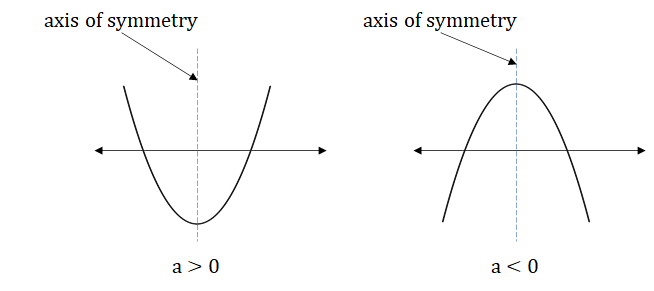​​​​​​​

The value of discriminant (D) determined whether the curve cuts the x-axis or not.

Case 1 If D < 0, then there is no real value of x for which f(x) = 0.
Hence, the graph does not touch the x-axis and lies completely above or below x-axis depending on the value of a.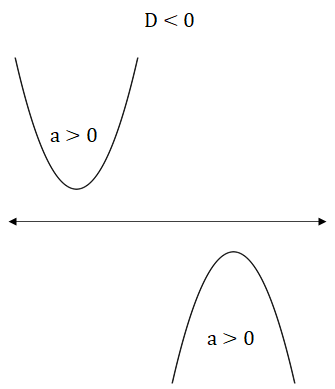​​​​​​​

Case 2: If D = 0, then both the real roots are same.
Hence, the graph touches the x-axis at exactly one point.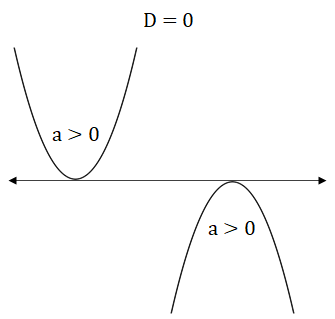​​​​​​​

Case 3: If D > 0, then both the real roots are distinct.
Hence, the graph cuts the x-axis at exactly two different points.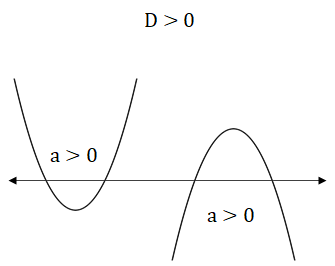​​​​​​​

Example: f(x) is a quadratic expression such that the coefficient of x2 is positive. If the roots of f(x) = 0 lie in the interval (–1, 1), then which of the following is necessarily true?
(a) f(1) > 0 & f(-1) > 0    (b) f(1) > 0 & f(-1) < 0    (c) f(1) < 0 & f(-1) > 0    (d) f(1) < 0 & f(-1) < 0

Solution:
Since the coefficient of x2 is positive, the graph of the quadratic expression will be upward facing.

Since the roots lie between -1 and 1, the graph will cut the x-axis at two points between x = -1 and x = 1.

The graph would look something like this.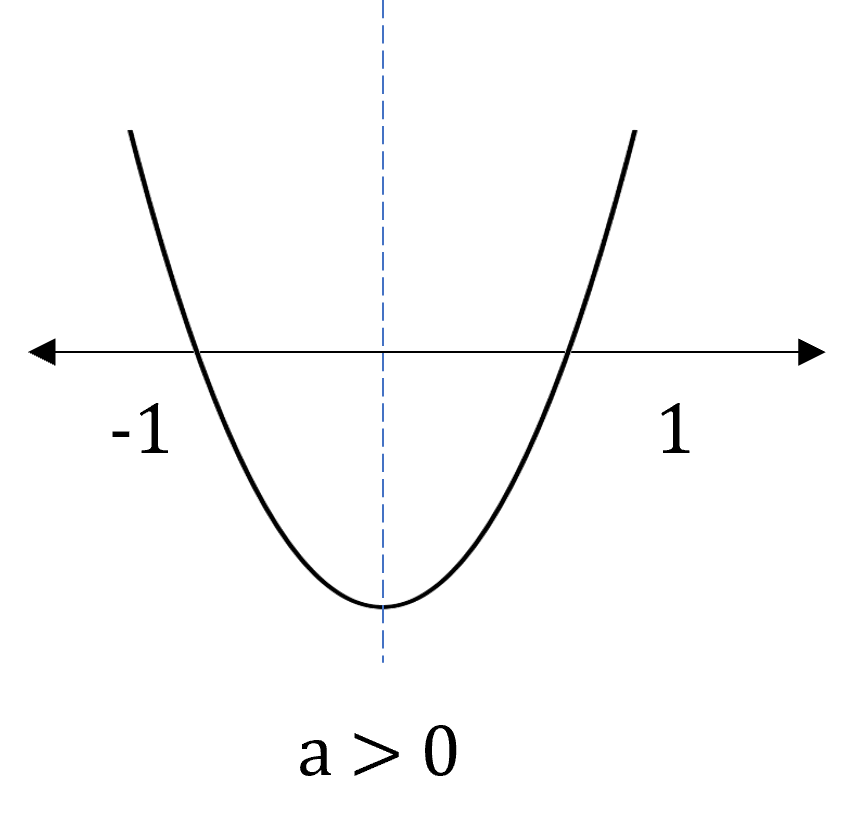​​​​​​​

We can see that f(1) and f(-1), both are greater than ‘0’.

Hence, option 1.

MAXIMUM / MINIMUM VALUE OF A QUADRATIC FUNCTION

Let f(x) = ax2 + bx + c (Quadratic function)

Case 1: a > 0
If a > 0 , the graph for the quadratic expression will be upward facing.

∴ The maximum value of the quadratic function will be ∞.

To calculate the minimum value of the function we need to first calculate the value of x for which f(x) will be minimum.

Since the curve is symmetric, the two roots lie at the same distance on either side from the point of symmetry.

Therefore, the value of x when f(x) is minimum is exactly in the middle of the two roots.

∴ x = $\frac{{\mathrm{x}}_{1}+{\mathrm{x}}_{2}}{2}$ = $\frac{-\mathrm{b}/\mathrm{a}}{2}$ = $-\frac{\mathrm{b}}{2\mathrm{a}}$

Therefore, at x = -b/2a, f(x) is least possible.

The least value of f(x) = $f\left(\frac{-\mathrm{b}}{2\mathrm{a}}\right)$ = $\mathrm{a}×{\left(\frac{-\mathrm{b}}{2\mathrm{a}}\right)}^{2}$ + $\mathrm{b}×\left(\frac{-\mathrm{b}}{2\mathrm{a}}\right)$ + c = $\frac{4\mathrm{ac}-{\mathrm{b}}^{2}}{4\mathrm{a}}$

Case 2: a < 0
If a < 0 , the graph for the quadratic expression will be downward facing.

∴ The minimum value of the quadratic function will be -∞.

To calculate the maximum value of the function we need to first calculate the value of x for which f(x) will be maximum.

Since the curve is symmetric, the two roots lie at the same distance on either side from the point of symmetry.

Therefore, the value of x when f(x) is maximum is exactly in the middle of the two roots.

∴ x = $\frac{{\mathrm{x}}_{1}+{\mathrm{x}}_{2}}{2}$ = $\frac{-\mathrm{b}/\mathrm{a}}{2}$ = $-\frac{\mathrm{b}}{2\mathrm{a}}$

Therefore, at x = -b/2a, f(x) is highest possible.

The highest value of f(x) = $f\left(\frac{-\mathrm{b}}{2\mathrm{a}}\right)$ = $\mathrm{a}×{\left(\frac{-\mathrm{b}}{2\mathrm{a}}\right)}^{2}$ + $\mathrm{b}×\left(\frac{-\mathrm{b}}{2\mathrm{a}}\right)$ + c = $\frac{4\mathrm{ac}-{\mathrm{b}}^{2}}{4\mathrm{a}}$

Note: You don’t need to remember the formula for highest value of f(x). Just remember that f(x) is highest at x = -b/2a and substitute the value of x in f(x) to find its highest value.

If the roots of a quadratic equation ax2 + bx + c = 0 are α and β, then:

Form a Quadratic Equation whose roots are k more than original equation

Quadratic equation whose roots are α + k and β + k will be obtained by replacing x by x – k in the original equation
i.e., a(x - k)2 + b(x – k) + c = 0

Example: Form a quadratic equation whose roots are 2 more than the roots of 3x2 - 2x - 5 = 0.

Solution:
Here we will substitute x with x – 2.

∴ The new quadratic equation is 3(x - 2)2 - 2(x - 2) - 5 = 0

⇒ 3(x2 – 4x + 4) – 2x + 4 – 5 = 0

⇒ 3x2 – 14x + 11 = 0

Alternately,
Given, 3x2 - 2x - 5 = 0

⇒ 3x2 – 5x + 3x – 5 = 0

⇒ (x + 1)(3x - 5) = 0

∴ The roots of the given equation are -1 and 5/3

⇒ Roots of the new quadratic equation are -1 + 2 = 1 and 5/3 + 2 = 11/3

∴ The new equation is (x - 1)(x – 11/3) = 0

⇒ (x - 1)(3x - 11) = 0

⇒ 3x2 – 14x + 11 = 0

Form a Quadratic Equation whose roots are k less than original equation

Quadratic equation whose roots are α - k and β - k will be obtained by replacing x by x + k in the original equation
i.e., a(x + k)2 + b(x + k) + c = 0.

Example: Form a quadratic equation whose roots are 2 less than the roots of 3x2 - 2x - 5 = 0.

Solution:
Here we will substitute x with x + 2.

∴ The new quadratic equation is 3(x + 2)2 - 2(x + 2) - 5 = 0

⇒ 3(x2 + 4x + 4) – 2x - 4 – 5 = 0

⇒ 3x2 + 10x + 3 = 0

Alternately,
Given, 3x2-2x-5=0

⇒ 3x2 – 5x + 3x – 5 = 0

⇒ (x + 1)(3x - 5) = 0

∴ The roots of the given equation are -1 and 5/3

⇒ Roots of the new quadratic equation are -1 - 2 = -3 and 5/3 - 2 = -1/3

∴ The new equation is (x – (-3))(x – (-1/3)) = 0

⇒ (x + 3)(3x + 1) = 0

⇒ 3x2 + 10x + 3 = 0

Form a Quadratic Equation whose roots are k times that of the original equation

Quadratic equation whose roots are kα and kβ will be obtained by replacing x by x/k in the original equation
i.e., a(x/k)2 + b(x/k) + c = 0

Example: Form a quadratic equation whose roots are three times the roots of 3x2-2x-5=0.

Solution:
Here we will substitute x with x/3.

∴ The new quadratic equation is 3(x/3)2 - 2(x/3) - 5 = 0

⇒ x2/3 - 2x/3 - 5 = 0

⇒ x2 - 2x - 15 = 0

Alternately,
Given, 3x2-2x-5=0

⇒ 3x2 – 5x + 3x – 5 = 0

⇒ (x + 1)(3x - 5) = 0

∴ The roots of the given equation are -1 and 5/3

⇒ Roots of the new quadratic equation are -1 × 3 = -3 and 5/3 × 3 = 5

∴ The new equation is (x – (-3))(x – 5) = 0

⇒ (x + 3)(x – 5) = 0

⇒ x2 - 2x - 15 = 0

Form a Quadratic Equation whose roots are 1/k times that of the original equation

Quadratic equation whose roots are α/k and β/k will be obtained by replacing x by kx in the original equation
i.e., a(kx)2 + b(kx) + c = 0.

Example: Form a quadratic equation whose roots are half the roots of 3x2-2x-5=0.

Solution:
Here we will substitute x with 2x.

∴ The new quadratic equation is 3(2x)2 - 2(2x) - 5 = 0

⇒ 12x2 - 4x - 5 = 0

Alternately,
Given, 3x2-2x-5=0

⇒ 3x2 – 5x + 3x – 5 = 0

⇒ (x + 1)(3x - 5) = 0

∴ The roots of the given equation are -1 and 5/3

⇒ Roots of the new quadratic equation are -1 ÷ 2 = -1/2 and 5/3 ÷ 2 = 5/6

∴ The new equation is (x – (-1/2))(x – 5/6) = 0

⇒ (2x + 1)(6x - 5) = 0

⇒ 12x2 - 4x - 5 = 0

• Form a Quadratic Equation whose roots are reciprocal of the roots of original equation

Quadratic equation whose roots are 1/α and 1/β will be obtained by replacing x by 1/x in the original equation
i.e., a(1/x)2 + b(1/x) + c = 0.

• Form a Quadratic Equation whose roots are equal in value but opposite in sign of the roots of original equation

Quadratic equation whose roots are -α and -β will be obtained by replacing x by -x in the original equation
i.e., a(-x)2 + b(-x) + c = 0.

Common roots of two Quadratic Equations

Let f(x) = 0 and g(x) = 0 be two quadratic equations in x such that they have a common root(s). You would be asked to find the common root(s).

Let’s say α is the common root of the two equations.

Instead of finding roots of f(x) and g(x), we can simply find the roots of f(x) = g(x), i.e., f(x) – g(x) = 0

Note: If f(α) = 0 and g(α) = 0, the f(α) - g(α) will also be equal to 0 but the converse is not true.

i.e., solving for f(x) – g(x) = 0, will give us two roots. Now we need to check for which of these two roots both f(x) and g(x) are equal to ‘0’.

Step 1: Find f(x) - g(x)

Step 2: Find the root(s) of f(x) - g(x) = 0

Step 3: Substitute the roots of f(x) - g(x) = 0 in f(x) and g(x). If the roots satisfy both the equations, then they are the common roots.

Example: The number of roots common between the two equations x3 - 6x2 + 11x - 6 = 0 and x3 + 2x2 - x - 2 = 0 is:
(1) 0            (2) 1            (3) 2             (4) 3

Solution:
f(x) = x3 - 6x2 + 11x - 6 = 0   ...(1)
g(x) = x3 + 2x2 - x - 2 = 0   ...(2)

Equating equations f(x) and g(x), we get,

⇒ x3 - 6x2 + 11x - 6 = x3 + 2x2 - x - 2

⇒ 8x2 – 12x + 4 = 0

⇒ 2x2 – 3x + 1 = 0

⇒ (2x – 1)(x – 1) = 0

∴ x = 1/2 or x = 1

Only for x = 1, both f(x) = 0 and g(x) = 0

Hence, option 2.

Example: The number of roots common between the two equations x3 + 3x2 + 4x + 5 = 0 and x3 + 2x2 + 7x + 3 = 0 is:
(1) 0            (2) 1            (3) 2             (4) 3

Solution:
f(x) = x3 + 3x2 + 4x + 5 = 0   ...(1)
g(x) = x3 + 2x2 + 7x + 3 = 0   ...(2)

Step 1: Equating equations f(x) and g(x), we get,

⇒ x3 + 3x2 + 4x + 5 = x3 + 2x2 + 7x + 3

⇒ x2 – 3x + 2 = 0

⇒ (x – 1)(x – 2) = 0

∴ x = 1 or x = 2

Since both x = 1 and x = 2, do not satisfy either (1) or (2), there exists no common roots for equations (1) and (2).

Hence, option 1.

Example: Find the value(s) of ‘m’, so that the equations x2 – x – 12 = 0 & mx2 + 10x + 3 = 0 have exactly one root in common.
(a) 3                      (b) -43/16        (c) 4                    (d) Either (a) or (b)

Solution:
f(x) = x2 – x – 12
g(x) = mx2 + 10x + 3

Solving for f(x)
⇒ (x + 3)(x - 4) = 0

⇒ x = -3 or 4

The common root will be either -3 or 4

Case 1: -3 is the common root

⇒ g(x) = mx2 + 10x + 3 = 0

⇒ 9m – 30 + 3 = 0

⇒ m = 3

Case 2: 4 is the common root

⇒ g(x) = mx2 + 10x + 3 = 0

⇒ 16m + 40 + 3 = 0

⇒ m = -43/16

Hence, option 4.

HIGHER DEGREE POLYNOMIALS

A Polynomial Expression of degree n can be written as:

anxn + an-1xn-1 + an-2xn-2 + ... + a2x2 + a1x + a0

A Polynomial Equation of degree n can be written as:

anxn + an-1xn-1 + an-2xn-2 + ... + a2x2 + a1x + a0 = 0

The above polynomial equation has 'n' roots / solutions / zeroes.

Sum of all the roots of the equation =

Product of all the roots of the equation =

## Feedback

Help us build a Free and Comprehensive Preparation portal for various competitive exams by providing us your valuable feedback about Apti4All and how it can be improved.